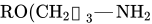# 测试SVG与公式(plain svg)

${C}_{z}^{-M}\frac{\prod _{m=1}^{{M}_{o}}\left(z-{z}_{m}^{0}\right)}{\prod _{m=1}^{{M}_{o}}\left(z-{z}_{m}^{\infty }\right)}$

svg在缺少字体的情况下无法正确显示

png版本